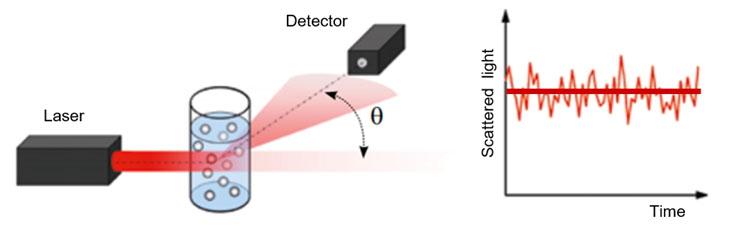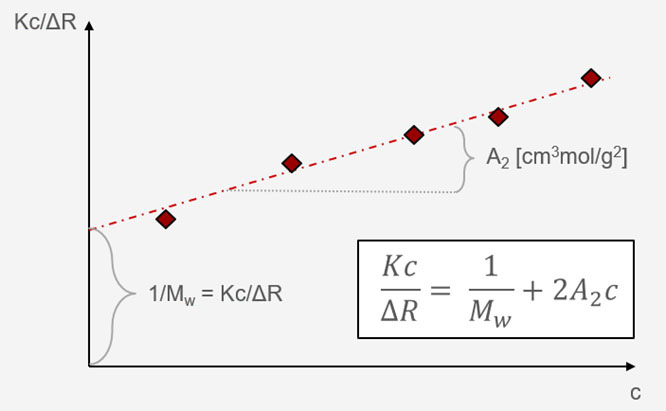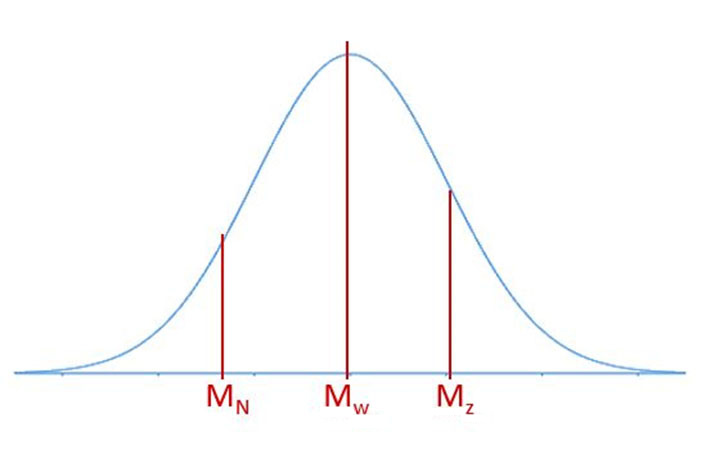0 Rates

# Molecular mass measurement using static light scattering

Molecular mass and molecular weight are crucial parameters in the characterization of a wide variety of sample groups, such as proteins (physical instability, assessment of functionality), polymers (melting point, elasticity) or nanoparticles (aggregation, core-shell structure analysis). Static light scattering (SLS) offers an easy-to-use solution to determine absolute molecular mass and is usually possible with a multi-purpose instrument also providing further means of characterization, such as determination of the isoelectric point or particle size.

## Introduction

The description of the mass of an atom or a molecule was an intriguing question at the dawn of modern science. Several definitions were formulated, many of which are still used today. Table 1 summarizes the most important ones, together with the units to which they correspond. Even though the definitions and units are different, since 1⁄12 of the mass of 1 mole C12 atoms was set by definition as 1 g, the molar mass, as well as the relative and absolute molecular mass, are numerically identical (1 Da = 1 g/mol).

 Name Unit Description Molar mass g/mol Mass (g) per amount of a substance (mol) Relative molecular mass - Mass of a molecule relative to 1/12 of the C12 atom. Absolute molecular mass Da, u Mass of a molecule Molecular weight amu, g Mass of 1 molecule of a substance

Table 1 - Summary of different terms commonly used for the description of molecule mass and their definitions

Molecular mass constitutes crucial information in the basic characterization of a number of sample groups, such as proteins, polymers or nanoparticles. In the case of proteins, it provides information about physical stability[A] and structure[B]. The molecular mass of a polymer is often understood as the chain length and determines physical properties such as melting point, viscosity, or elasticity. Nanoparticles are also often characterized by their molecular mass, to investigate possible shell-core structure, or aggregation.

While it is easy to calculate molecular mass for small particles, with macromolecules, such as proteins or polymers, it often becomes challenging. These molecules don’t have a uniform formula throughout their volume, and therefore different methods are used to calculate average molecular mass.

This article introduces the reader to molecular mass measurement by means of static light scattering and to evaluation of the Debye plot.

## Theoretical background to static light scattering

When light comes in contact with any particle, it is forced to deviate from a straight trajectory. This phenomenon is called scattering[C]. As the intensity of scattered light is proportional to the radius of the particle, this information can be used to draw a conclusion about the size of the particles in the dispersion.

The connection between scattering intensity and molecular mass is described in the Debye-Zimm equation, which is the theoretical basis for static light-scattering measurements:

$${Kc \over ∆R} = {1 \over M_w P(θ) } (1+2A_2 c + …)$$ (1)

Where c is the concentration of the particles, ΔR is the Rayleigh ratio of a reference solvent, K is an optical constant, Mw is the weight average molecular mass, P(θ) is the form factor and A2 is the 2nd virial coefficient.

The optical constant K is by definition equal to:

$$K=4π^2 ({dn \over dc})^2 {n_0^2 \over N_A λ_0^4 }$$ (2)

Where dn/dc is the refractive index increment, n0 is the refractive index of the solvent, NA is Avogadro’s constant and λ0 is the wavelength of the incident beam.

The evaluation route is different depending on the ratio of the wavelength and the particle size. If the particles are larger than roughly λ0/20, the angular dependent form factor P(θ) cannot be neglected, and it is required to determine the scattering intensity at multiple angles around the sample. The evaluation is then performed through the Zimm plot, which implies a double-extrapolation to zero concentration and zero scattering angle resulting in a characteristic rhomboid plot. As the angular information is available, it is also possible to extract the radius of gyration (Rg).

For particles with dimensions smaller than λ0/20, the angular dependent form factor can be neglected, and the Zimm-Debye equation simplifies to:

$${Kc \over ∆R}={1 \over M_w} (1+2A_2 c )$$ (3)

In this case, the evaluation is performed through the Debye plot which marks Kc/ΔR over the concentration (s. Section 6). This approach is inherent to the static light-scattering method, which is explained in detail below. As the scattering intensity is uniform around the particles, the radius of gyration cannot be determined using laser-based instruments. If the radius of gyration is of interest, an X-ray source is required (see also small-angle X-ray scattering, SAXS)[D].

## Static light scattering: Technical realization

During a static light scattering measurement, a coherent laser beam is directed through the sample and the scattering intensity is measured at a fixed angle of typically 90°, as this angle provides the best signal-to-noise ratio for weakly scattering samples. (s. Figure 1)Figure 1 - Typical measurement setup of a static light-scattering measurement

As the determination of molecular mass requires an extrapolation-to-zero concentration, a concentration series has to be prepared and measured. Additionally, in order to subtract the scattering background signal of the solute, the pure solvent also has to be measured. Subsequently, to situate the measured data on an absolute intensity scale, the scattering intensity of a known scatterer is also measured (typically toluene, see also Section 5.1. Molecular mass Mw).

## Static light scattering: Input parameters

### Rayleigh ratio

The Rayleigh ratio, ΔR, is the scattered intensity of a sample on an absolute scale. This absolute intensity is required to calculate absolute molecular mass. Light-scattering instruments, however, only provide a count rate on an arbitrary scale. This count rate depends on instrument parameters, such as the laser intensity, the quantum efficiency of the detector, the size of the scattering volume, the solid angle of the detector, and the accuracy of the alignment. It is impractical to determine all these parameters separately, therefore commercial instruments (e.g. Anton Paar’s Litesizer 500) are calibrated using a known solvent as reference, most often toluene or benzene. Since the Rayleigh ratio (= the absolute scattering intensity) of the reference solvent is known, it is possible to define the intensities of the samples on an absolute scale.

### Refractive index increment

The refractive index increment (dn/dc) describes the change of refractive index with concentration. This constant value is characteristic to specific material solvent pairs. While for often-used materials the refractive index increment is accessible via online libraries, for more exotic sample-solvent pairs an additional measurement using a refractometer with, optimally, the same wavelength as the light-scattering instrument, is required. Finding the correct refractive index increment is further complicated by the fact that, in the case of polymers, the chain length also influences the value of dn/dc. Careful determination of the refractive index increment is key to precise determination of molecular weight.

### Refractive index of the solvent

The refractive index of the most typical solvents is available in online libraries; however, as the refractive index is dependent on the wavelength of the laser source, it is advisable to use a refractive index which matches the wavelength of the light-scattering instrument. Litesizer 500 offers a refractive index determination at the exact wavelength of the light source also used for static light-scattering measurement.

## Evaluation

From the acquired concentration-scattering intensity data pairs, the Debye plot is constructed, where Kc/ΔR is plotted against the concentration. The intercept of the fitted line gives the molecular mass, while the slope corresponds to the 2nd virial coefficient. (s. Figure 2)Figure 2 - Evaluation of the static light-scattering measurement using the Debye plot

### Molecular mass Mw

The molecular mass of strictly monodisperse samples, such as a protein monomer dispersion, can be analyzed as follows. For polymer dispersion, or polydisperse protein mixtures, however, molecular mass is better represented by a distribution curve. In such cases, it is important to be mindful about the fact that the resulting molecular mass from a static light-scattering measurement will correspond to the weight average of the distribution (Mw). This is the average value which is closest to the middle of the distribution, as shown in Figure 3. In comparison, the other two most often-used average values are the z-average (corresponding to the radius of gyration), which represents the highest portion of the distribution, and the number average, which is closer to the lowest portion of the curveFigure 3 - An exemplary bell curve distribution with estimated positions of the different average values

### Second virial coefficient

Besides the molecular mass, the method of static light scattering also determines the second virial coefficient (A2). This parameter is a thermodynamic term, which describes the particle-solvent and particle-particle interactions. In general, if the second virial coefficient is positive, particle-solvent interactions are energetically favorable, while if the term is negative, particle-particle interactions are favored. However, the exact interpretation of the thermodynamic term differs for different sample groups.

In the case of protein or nanoparticle dispersions, the 2nd virial coefficient can be used to predict aggregation in different solutes. While a negative value suggests that aggregation is likely, a positive value reflects good particle-solvent interactions, and therefore stability. A2 is dependent on the ionic strength (e.g. salt concentration) as well as the pH value and the temperature of the dispersion.

For polymer solutions, based on the sign of the 2nd virial coefficient, we differentiate between good, bad and theta solvents. If A2 is positive, it means that the polymer-solvent interaction is favorable and therefore the polymer coil will expand. Contrarily, if the term is negative, polymer-polymer interaction is preferred, and therefore the coil contracts. If the 2nd virial coefficient is exactly zero, then we refer to the solvent as a theta solvent. As the 2nd virial coefficient is temperature-dependent, it is also called the theta state. Determining the theta state of a polymer-solvent system is beneficial for further characterization of the sample. In the theta state, the polymer behaves exactly as an ideal chain, which makes it easier to determine further parameters, such as the root mean square end-to-end distance.

## Summary

Molecular mass determination by means of static light scattering is a versatile tool for the characterization of several sample groups, such as proteins, polymer dispersion or nanoparticles. It enables not only the precise determination of the molecular weight, but also provides information about the dispersion stability through the second virial coefficient. The method of static light scattering is typically available in instruments which also offer determination of particle size via dynamic light scattering[E] and stability / zeta potential analysis via electrophoretic light scattering[F], such as Anton Paar’s Litesizer 500